Next: The Hartree-Fock-Bogolyubov mean fields Up: The Skyrme Hartree-Fock-Bogolyubov equations Previous: Spherical symmetry

## The Hartree-Fock-Bogolyubov energy

In the Skyrme-HFB approximation, the total energy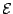of a nucleus is given as a sum of kinetic, Skyrme, pairing and Coulomb terms: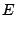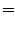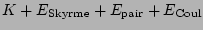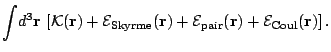(16)

The derivation of the energy is explained in detail in several articles (see Refs. [3,11] for the HF energy and  for the HFB case). The expression of the Skyrme force is given in section 3.

In equation (17), the kinetic energy of both neutrons and protons is given by isoscalar kinetic density. The neutron and proton masses being approximated by their average value, and one has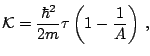(17)

where the factor in parentheses takes into account the direct part of the center-of-mass correction . Since we only consider even-even nuclei, the Skyrme part of the energy functional is time even, it can be written as a sum of isoscalar (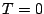) and isovector (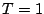) parts (see e.g. Ref. ) or as a sum on isoscalar, neutrons and protons densities,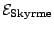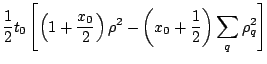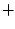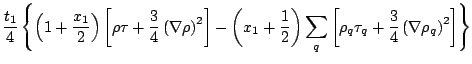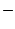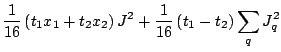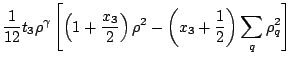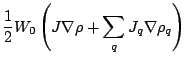(18)

Index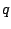stands for neutrons and protons while the absence of index indicates the total (isoscalar) density. Using the same notations for the densities, the pairing energy density reads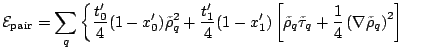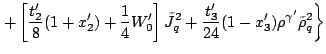(19)

In this last expression we have added a prime to the parameters of the interaction. Although the derivation of the general Skyrme energy density functional is based on a unique force, its effective nature justifies the use of different sets of parameters in the particle-hole and particle-particle channels. The prime indices anticipates this possibility which is discussed in section 3.

The energy density defined in (17) involves a Coulomb term for protons. This term contains a direct part which can be expressed using the charge density and leads to a local field after variation of the energy and an exchange part which would lead to a non local potential. Both parts deserve a special discussion. The direct part of the Coulomb energy depends on the charge density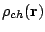and reads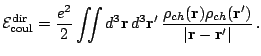(20)

We use the point proton density to simplify this expression, i.e. the charge density is replaced by the proton density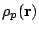. Nevertheless, the proton form factor is taken into account when we calculate the charge radius of the nucleus (see eq. (57)). The exchange part of the Coulomb energy leads to a non local term and is treated with the Slater approximation. This approximation means that we keep only the first term of the density matrix expansion in the local density approximation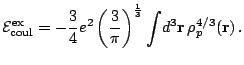(21)

The error introduced by this approximation has been estimated by studying the next order term  or more recently by comparison with the exact treatment of the Coulomb energy . The Slater approximation seems to have little consequence on the nuclei bulk properties although some significant effects can be expected on the position of the proton drip-line and the Coulomb displacement energy of single particle levels. Finally, the Coulomb contributions to the pairing energy and fields are not included in the HFBRAD code.Next: The Hartree-Fock-Bogolyubov mean fields Up: The Skyrme Hartree-Fock-Bogolyubov equations Previous: Spherical symmetry
Jacek Dobaczewski 2005-01-23